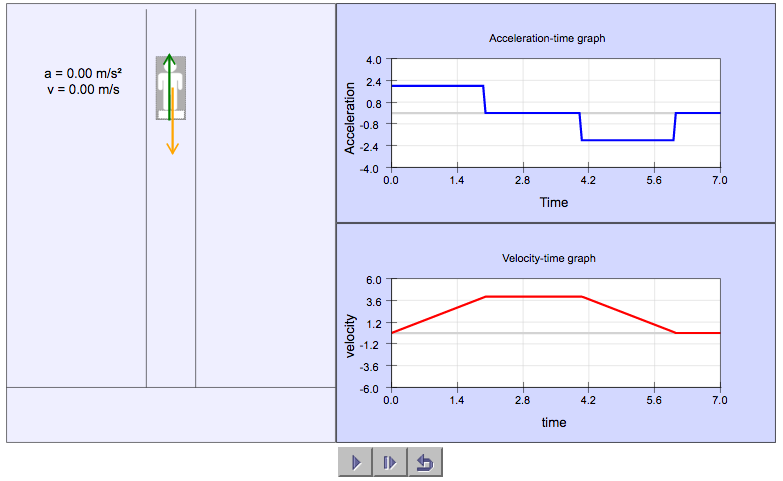# Man in Elevator SimulationIn this simulation, students can observe the variation of the normal contact force (N) and its effect on acceleration and velocity as an elevator moves upward.

Questions for students to work on can include:

1. Express the acceleration as a function of Normal Contact Force (N), Weight (W) and mass of the man.
2. Determine the distance travelled by the elevator.
3. Predict how the forces, acceleration and velocity will differ if the elevator was moving down instead.